Medizinische Klinik für Gastroenterologie, Infektiologie und Rheumatologie (einschl. Arbeitsbereich Ernährungsmedizin) CBF Institut für Klinische Physiologie / AB Ernährungsmedizin

The electrical impedance of epithelia
Alfred H. Gitter

 Part  I:  The semicircle model of epithelial impedance Part II:  Model with apical and basolateral membrane  References

Part I: The semicircle model of epithelial impedance

Equivalent electrical circuit

The electrical properties of an epithelium may, in the simplest way, be represented by the model of an "RC-element", which consists of an ohmic resistance (RP) and a capacitor (CP) in parallel. If the ohmic properties of subepithelial tissue or, in cell cultures, that of support material are to be considered, a series resistance (RS) is added (Fig. 1).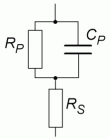Fig. 1   Simple equivalent circuit of epithelium plus subepithelial tissues The epithelium is represented by an RC-element, consisting of an ohmic resistance RP and a capacitance CP. RS is the series resistance of the cell culture support or, in native tissue, of the subepithelial tissue.

With impedance spectroscopy, the permeability for alternating electric current is investigated and the components of the circuit shown in Fig. 1 can be determined.

Electrical impedance

Let us consider an alternating voltage (v), which changes sinusoidally with time (t),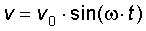, (1)

where vo is the amplitude and w the angular velocity. Since the argument of the sine is given in radians, the angular velocity w equals 2× p × f, where f is the frequency of the alternating voltage. Likewise we define alternating current (i) by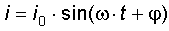, (2)

where the phase angle j introduces a lag between v and i.

The apparent opposition in an electrical circuit composed of ohmic resistors and capacitances to the flow of i (analogous to the electrical resistance to a direct current) is called electrical impedance (Z). It is given as complex number, in order to simplify the rules for computations.

The impedance of an ohmic resistance is R. For a capacitance C is Z = 1 / (j× w × C), where j denotes the imaginary unit.

The impedance of the equivalent electrical circuit

The impedance ZP of an ohmic resistor (resistance RP) and a capacitance CP (reactance 1 / (w × CP)) in parallel can be derived from Kirchhoff's laws,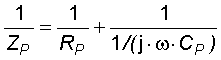. (3)

Rearrangement of the equation yields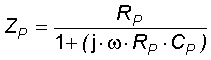. (4)

If an additional ohmic resistance RS is put in series to the RC-element (Fig. 1) we get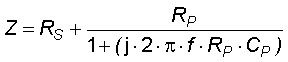. (5)

where w is replaced by 2× p × f. The real (Zre) and the imaginary part (Zim) of the complex impedance Z are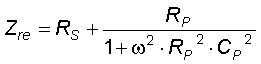(6)

and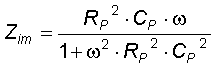, (7)

respectively. Taken together, equations 6 and 7 yield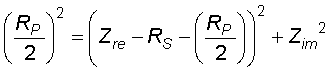. (8)

If RP, CP and RS are held constant and w is a variable parameter (0 Ł w Ł Ą), this equation describes a semicircle in the plane of complex numbers.

Graphic demonstration in a Nyquist plot

The parametric equation of impedance as a function of frequency can be graphed in the plane of complex numbers (Fig. 2). Abscissa and ordinate show real (Zre) and imaginary part (Zim) of the impedance, respectively. The diagram is called a Nyquist plot. (The phase angle of 90° between capacitive and ohmic currents is represented by the orthogonality of the axes of the coordinate system.)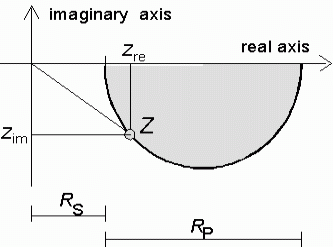Fig. 2   Nyquist plot Impedance is graphed in the plane of complex numbers, which is divided into four quarters (quadrants) by the real and imaginary axes. The impedance locus of the circuit shown in Fig. 1 forms a circular arc in the IV. quadrant of the coordinate system. RS and RP can be derived from the intersections with the abscissa of the extrapolated impedance curve.

From equation 8 follows that in a Nyquist plot the impedance locus of the equivalent electrical circuit shown in Fig. 1 describes a circular arc with its center on the abscissa.

The intersections of the impedance curve extrapolated to the abscissa are at (RS, 0) for f ® Ą and (RS + RP , 0) for f ® 0. Since Zre is a monotonously declining function of frequency f, the points on the impedance curve are ordered counterclockwise in the sequence of decreasing frequency.

Part II: Model with apical and basolateral membrane

The most simple electrical model of an epithelium with apical and basolateral cell membranes and considerable paracellular ion permeability is shown in Fig. 3. Here, the transmural impedance is the sum of the transepithelial impedance and the resistance of the material on which the epithelium grows (Rsub). The transepithelial admittance (the reciprocal of impedance) is the sum of transcellular and paracellular admittance.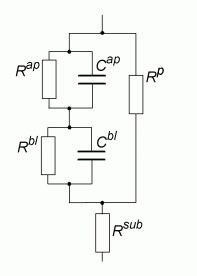Fig. 3   Equivalent circuit of an epithelium (2 membranes and paracellular pathway) Apical and basolateral cell membranes are represented by a resistance and a capacitance in parallel (Rap and Cap; Rbl and Cbl). The paracellular pathway is represented by the resistance Rp. The series resistance of the support filter (if cell cultures are studied) or the subepithelial cells (if native tissue is studied) is Rsub.

The transcellular impedance is the sum of the impedances of apical and basolateral cell membranes. Each membrane may be described by a resistance and a capacitance in parallel (Rap and Cap or Rbl and Cbl, respectively). Moreover, in parallel to the transcellular pathway is the paracellular, which is represented by a resistance (Rp). Thus we derive at the transmural impedance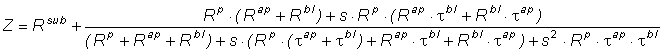(9)

where s = j × 2 × p × f is the imaginary unit times the angular frequency of the clamp current; t ap and t bl are the time constants of apical and basolateral membranes, (Rap × Cap) and (Rbl × Cbl), respectively.

Equation 9 contains 6 parameters (Rsub, Rap, Rbl, Rp, t ap, t bl), two of which are frequency dependent (t ap und t bl). However, only 5 independent parameters can be determined in one transepithelial impedance recording, if no other relations of the parameters hold.

References

• Gitter AH, Bendfeldt K, Schulzke JD, Fromm M (2000) Trans-/paracellular, surface/crypt, and epithelial/subepithelial resistances of mammalian colonic epithelia. Pflügers Arch. 439(4): 477-482. [Medline Abstract] [Full text] [Related articles]
• Gitter AH, Fromm M, Schulzke JD (1998) Impedance analysis for the determination of epithelial and subepithelial resistance in intestinal tissues. J. Biochem. Biophys. Methods 37: 35-46 [Medline Abstract]
• Gitter AH, Schulzke JD, Sorgenfrei D, Fromm M (1997) Ussing chamber for high-frequency transmural impedance analysis of epithelial tissues. J. Biochem. Biophys. Methods 35: 81-88 [Medline Abstract]
• Hegel U, Fromm M (1990) Electrical measurements in large intestine (including colon, rectum, caecum). Methods Enzymol. 192: 459-484. [Medline Abstract]
• Fromm M, Schulzke JD, Hegel U (1985) Epithelial and subepithelial contributions to transmural electrical resistance of intact rat jejunum, in vitro. Pflügers Arch. 405: 400-402. [Medline Abstract]

 Charité  > Campus Benjamin Franklin  > Inst. Clin. Physiology  > Topics  > impedance:Top